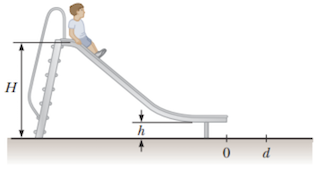# Problem: A boy starts at rest and slides down a frictionless slide as shown in the figure. The bottom of the track is a height h above the ground. The boy then leaves the track horizontally, striking the ground at a distance d as shown. Using energy methods, determine the initial height H of the boy above the ground in terms of h and d.

🤓 Based on our data, we think this question is relevant for Professor Efthimiou's class at UCF.

###### Problem Details

A boy starts at rest and slides down a frictionless slide as shown in the figure. The bottom of the track is a height h above the ground. The boy then leaves the track horizontally, striking the ground at a distance d as shown. Using energy methods, determine the initial height H of the boy above the ground in terms of h and d.What scientific concept do you need to know in order to solve this problem?

Our tutors have indicated that to solve this problem you will need to apply the Solving Projectile Motion Using Energy concept. You can view video lessons to learn Solving Projectile Motion Using Energy. Or if you need more Solving Projectile Motion Using Energy practice, you can also practice Solving Projectile Motion Using Energy practice problems.

How long does this problem take to solve?

Our expert Physics tutor, Julia took 7 minutes and 14 seconds to solve this problem. You can follow their steps in the video explanation above.

What professor is this problem relevant for?

Based on our data, we think this problem is relevant for Professor Efthimiou's class at UCF.

What textbook is this problem found in?

Our data indicates that this problem or a close variation was asked in Physics for Scientists and Engineers - Serway Calc 9th Edition. You can also practice Physics for Scientists and Engineers - Serway Calc 9th Edition practice problems.